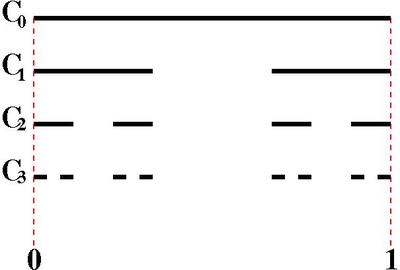# Cantor Set

Alignments to Content Standards: A-SSE.B.4

In this task we will investigate an interesting mathematical object called the Cantor Set. It is a simple example of a fractal with some pretty weird properties. Here is how we construct it: Draw a black interval on the number line from 0 to 1 and call this set $C_0$. Create a new set called $C_1$ by removing the “middle third” of the interval, i.e. all the numbers between $\frac13$ and $\frac23$. So $C_1$ consists of two black intervals of length one-third. We continue by removing the middle third of each of the two remaining intervals and calling this set $C_2$. We then take out the middle third of each remaining black interval to create $C_3$ and so on (see diagram below).1. How many black intervals are in $C_0$, $C_1$, $C_2$, $C_3$, $\ldots$ , $C_{10}$?
2. Add up the total length of the pieces that are removed at each stage. We can think of this as the total length of the ”gaps” between the black intervals. What is the total length of the gaps in $C_0$, $C_1$, $C_2$, $C_3$, $\ldots$, $C_{10}$?
3. If we continued to remove more and more middle thirds, how much length of the original interval would we eventually remove? Are there any points that would be left?

Note: As a thought experiment, imagine we could continue the middle third removal forever. There are still some numbers that would never get removed like $0$, $\frac13$, $\frac23$, $1$ etc. The set of numbers that will never be removed is called the Cantor Set and it has some amazing properties. For example, there are infinitely many numbers in the Cantor Set (even uncountably many numbers), but it contains no intervals of numbers and its total length is zero.

## IM Commentary

This task leads to the generation of finite geometric series with a common ratio less than one as a means to explore properties of the Cantor Set. The Cantor Set is a fascinating set with many intriguing properties. It contains uncountably many points, which means that there are “as many” points in it as on the real line, yet the set contains no intervals of real numbers and it has length zero. All that is necessary to show that it has length zero is to look at what happens to a geometric series as we add more and more terms.

The purpose of this task is to use finite geometric series to investigate an amazing mathematical object that might inspire students' curiosity. The Cantor Set is an example of a fractal. A process similar to the one that created the Cantor Set can produce beautiful objects like the Sirpinski Carpet, http://en.wikipedia.org/wiki/Sierpinski_carpet, or the Menger Sponge, http://en.wikipedia.org/wiki/Menger_sponge.

This task could be used for instruction, to pique students' curiosity, or as part of a more involved project investigating fractals.

## Solution

1. At each step we are doubling the number of black intervals. So $C_0$ has 1 black interval, $C_1$ has 2 black intervals, $C_2$ has $2^2 = 4$ black intervals, $C_3$ has $2^3 = 8$ black intervals, etc., and $C_{10} = 2^{10} = 1024$ black intervals.
• $C_0$ has no gaps, so the length is 0.
• $C_1$ has one gap of length $\frac13$.
• $C_2$ has two new gaps of length $\frac19$ each, so the total length is

$$\frac13 + \frac29 = \frac59$$
• We see that at each step we get a new gap for each black interval from the previous step. So $C_3$ has $2^2$ new gaps and they are $\frac13$ as long as in the previous step, so they are $\frac 1{27}$ long. Therefore, the total gap lengths for $C_3$ is

$$\frac13 + \frac{2^1}{3^2} + \frac{2^2}{3^3} = \frac{19}{27}.$$
• Following this pattern we find that the total gap length for $C_{10}$ is:

$$\frac13 + \frac{2^1}{3^2} + \frac{2^2}{3^3} \cdots + \frac{2^9}{3^{10}} = \frac13 + \frac13 \left( \frac23 \right) + \frac13 \left( \frac23 \right)^2 \cdots + \frac13 \left( \frac23 \right)^9$$

This is a finite geometric series with $a = \frac13, r = \frac23, \text{ and } n = 10$. Therefore, the final gap length is:

$$\left( \frac13 \right) \frac{1 - \frac{2}{3}^{10}}{1 - \frac{2}{3}} \approx .982658$$

Note that this number is already really close to 1, which is the length of our original interval.

2. If we could continue the “middle third removal” forever, it seems that eventually we would take out the entire length of the original interval from 0 to 1. However, in the final set, the Cantor Set, there are infinitely many points left, such as $0$, $\frac13$, $1$, and even weird ones like $\frac14$.